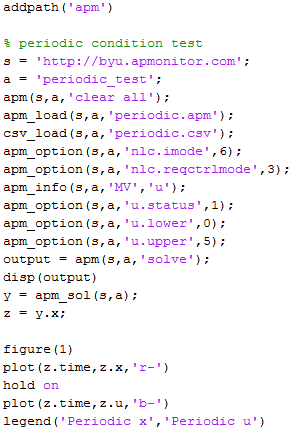Apps

## Apps.PeriodicBoundaryConditions History

May 28, 2014, at 10:49 PM by 107.188.175.164 -

#### Periodic Energy Storage

May 28, 2014, at 10:49 PM by 107.188.175.164 -May 28, 2014, at 10:48 PM by 107.188.175.164 -
Changed line 27 from:
to:
May 28, 2014, at 10:48 PM by 107.188.175.164 -
Changed lines 9-10 from:

The following example illustrates the use of the boundary condition. Scripts in MATLAB and Python are available below to recreate this solution.

to:

The following example illustrates the use of the boundary condition. Scripts in MATLAB and Python are available below to recreate this solution along with the model equations in APMonitor. Both MATLAB and Python scripts produce equivalent results.

Changed lines 13-16 from:
• MATLAB Script
to:

APMonitor ModelMATLAB Script

Changed line 19 from:
• Python Script
to:

Python ScriptA further example demonstrates a more complicated model for energy storage and retrieval. In this case, energy is stored during the first hours of the day when demand is lower. The power generation runs at a constant level while the energy storage is able to follow the cyclical demand. Energy storage is set to a periodic boundary condition to ensure that the beginning and end of the day have at least 100 units of stored energy. Scripts are available in both MATLAB and Python.

May 28, 2014, at 10:42 PM by 107.188.175.164 -
Changed lines 13-18 from:

(:table border=1 width=100%:) (:cell:) MATLAB (:cell:) Python (:cellnr:)(:cell:)(:tableend:)

to:
• MATLAB Script• Python ScriptMay 28, 2014, at 10:36 PM by 107.188.175.164 -
Changed lines 16-17 from:

(:cellnr:) (:cell:)to:

(:cellnr:)May 28, 2014, at 10:35 PM by 107.188.175.164 -
(:table border=1 width=100%:) (:cell:) MATLAB (:cell:) Python (:cellnr:) (:cell:)(:cell:)(:tableend:)